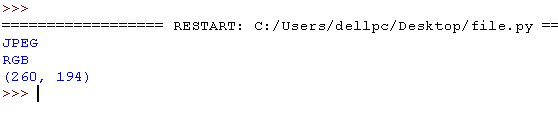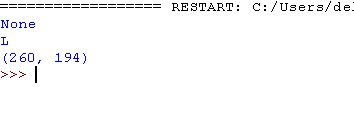×

Search anything:

# Working with images in Python with PIL like a Painter

#### Software Engineering PythonOne of the interesting feature working with python is it's ability of dealing with images in a very flexible manner. So let's see how to work with images in python. Python has it's own library for images called as -"PIL" (python image library).
And 'Pillow' is the updated version of PIL or python image library. This library helps in providing the python interpreter with image editing functionalities.It also forms the basis for simple image support in other Python libraries such as sciPy and Matplotlib.

Development contribution PIL was developed by Fredrik Lundh and several other contributors.It's developers include Secret Labs AB.Pillow was developed by Alex Clark and several other contributors.

Languages used Python image library is written in python and C (mainly).

Let's explore the updated version i.e Pillow:

NOTE If pip is not installed in your system and you get "pip is not recognised as an internal or external command" then either while downloading python select 'add pip to path' or add python bin path to your PC environment settings.Other way is installing pip via terminal (in Linux) by command-

``````sudo apt-get update
sudo apt-get install python-pip
``````

Installation

``````pip install pillow
``````

make sure that version used is suitable to python version you are using.

Importing Image module

``````from PIL import Image # use this line to use image module in our program
``````

Operations that can be done using PIL

## 1. Opening and display an image in Python

An image can be opened using the open() function of the image module like:

``````img = Image.open('<image path>')
``````

An image has multiple properties such as:

• Image format (can be jpeg, png, svg and many others)
``````img.format
``````
• Image mode (can be RGB, RGBA and others)
``````img.mode
``````
• Image Size: the height and width dimensions of the image
``````img.size
``````
• To open an image, use:
``````img.show()
``````

Code example:

``````from PIL import Image # importing image module

# open image using open function if image and python not in same directory then take care of path of image
img = Image.open('tomnjerry.jpg')

print(img.format) # display image format like jpeg,png etc
print(img.mode) # display image mode like RGB etc.
print(img.size) # display image size width and height

img.show() #showing image
``````

Result## 2. How to convert image to gray-scale in Python?

We can use the convert() function of the Image module for this like:

``````img = img.convert('L')
``````

Code:

``````from PIL import Image # importing image module

img = Image.open('tomnjerry.jpg').convert('L') # open image and converting it to gray-scale

print(img.format) # display image format like jpeg,png etc
print(img.mode) # display image mode like RGB etc.
print(img.size) # display image size width and height

img.show() # showing image
``````

Result## 3. How to save changes in an image?

By using save() we can do so like:

``````img.save('<path of image to be saved>')
``````

Code:

``````from PIL import Image#importing image module

img = Image.open('tomnjerry.jpg')#open image using open function if image and python not in same directory then take care of path of image

img.save('tomnjerry.png')#syntax is save(path,format) here format is optional if no format then it is used by the extension.

img.show()#showing image
``````

Result

On doing so another a new image file ('tomnjerry.png') is created and saved in same directory here .

## 4. Changing image angle by rotation

Image can be rotated using the rotate function of Image module like:

``````img = img.rotate(<angle>)
``````

See following code for this , we have used try catch block to deal with exceptions in our code

``````from PIL import Image#importing image module

def prog():
try:
img=Image.open('tomnjerry.jpg')
img=img.rotate(180)#angle is 180 by which we rotate our image here
img.save("rotated_image.jpg")
img1=Image.open('rotated_image.jpg')
img1.show()
except IOError:
pass
prog()
``````

Result## 5. Resizing an image

We can resize the image in python using resize() function ,keep in mind this function takes a tuple as an input (width,height)

``````img = img.resize((<height>, <widht>))
``````

Code:

``````from PIL import Image#importing image module

def prog():
try:
img=Image.open('tomnjerry.jpg')
img1=img.resize((200,80))
img1.show()
except IOError:
pass
prog()
``````

Result## 6. Pasting an image over another image

We can paste an image over another image by the help of paste() function.Suppose we want to paste an image img2 over another image img then we do so by command- img.paste(img2,(50,50)) , here the second argument can be a tuple of size 2 or 4.If size of tuple is 2 like (a,b) here a is distance from left while b is that from upper and tuple is of size 4 (a,b,c,d) i.e left,upper,right,lower.Make sure that size of image to be inserted inside should not exceed the original image.

``````from PIL import Image

def prog():
try:
img =Image.open("tomnjerry.jpg")#original image
img2=Image.open("duck2.jpg")#image to be pasted
img.paste(img2,(95,3))#tuple of size 2
img.save("new_picture.jpg")
img3=Image.open("new_picture.jpg")
img3.show()
except IOError:
pass

prog()
``````

Result## 7. Creating thumbnail

By the use of thumbnail() function we do so, also we can pass the tuple as argument to this function which helps to get the size .Keep in mind thumbnail fuction does not make new object for image but does modification in previous object itself.

``````from PIL import Image#importing image module

def prog():
try:
img=Image.open('tomnjerry.jpg')
img.thumbnail((90,90))
img.save("thumbnail.jpg")
img1=Image.open("thumbnail.jpg")
img1.show()
except IOError:
pass
prog()
``````

Result## 8. Transposing an image

We can get the mirror image of image by transpose(Image.FLIP_LEFT_RIGHT) function .Here Image.FLIP_LEFT_RIGHT is an integer value that corresponds to rotation such that image is transposed.

``````from PIL import Image#importing image module

def prog():
try:
img=Image.open('tomnjerry.jpg')
transpose_image=img.transpose(Image.FLIP_LEFT_RIGHT)
transpose_image.show()
except IOError:
pass
prog()
``````

Result## Question

#### How can one paste an image (img2) over another image(img)?

img.paste(img2, (50, 50))
img2.paste(img1, (50, 50))
img.join(img2,(50,50))
img.plac_over(img2,(50,50))
Answer explanation goes here. Only works with HTML

Conclusion: Here we see several ways of playing with images in python usinh PIL ie Python image library .Play with several other features of PIL and build cool stuff.